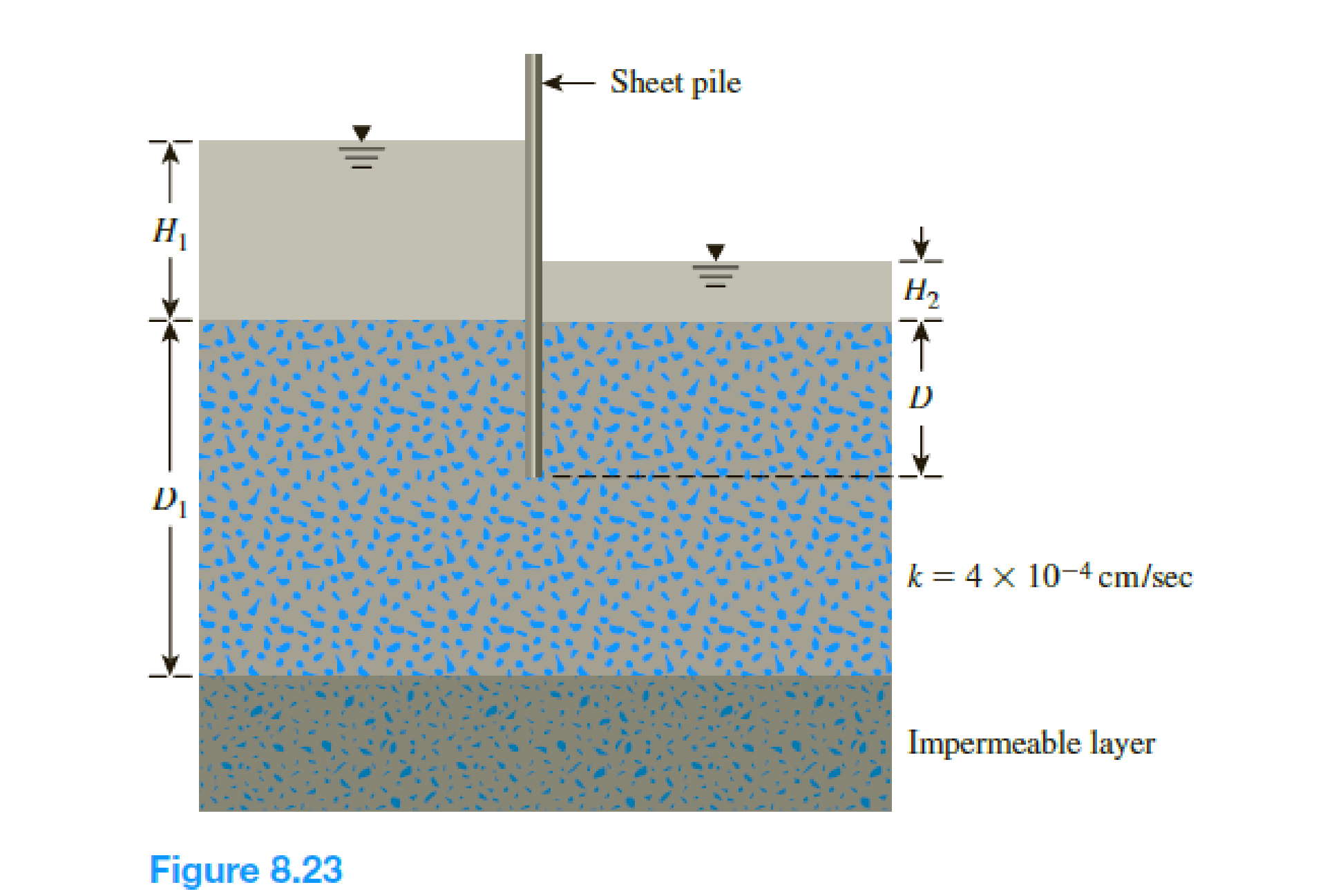Chapter 8, Problem 8.3PPrinciples of Geotechnical Enginee...

9th Edition
Braja M. Das + 1 other
ISBN: 9781305970939

Solutions

Chapter
SectionPrinciples of Geotechnical Enginee...

9th Edition
Braja M. Das + 1 other
ISBN: 9781305970939
Textbook Problem

Refer to Figure 8.23. Given: • H1 = 4 m • H2 = 1.5 m • D1 = 6 m • D = 3.6 m Calculate the seepage loss in m3/day per meter length of the sheet pile (at right angles to the cross section shown). Use Figure 8.12.To determine

Find the seepage loss in m3/day per meter length of the sheet pile.

Explanation

Given information:

The hydraulic conductivity of the permeable soil layer k is 4×104cm/sec.

The height of the water level H1 is 4 m.

The height of the water level H2 is 1.5 m.

The depth of permeable layer up to the end of sheet pile D is 3.6 m.

The depth of permeable layer D1 is 6 m.

Calculation:

Determine the head difference between the upstream and downstream using the relation.

H=H1H2

Substitute 4 m for H1 and 1.5 m for H2.

H=41.5=2.5m

Determine the ratio of S/T.

Here, S is the depth of penetration of the sheet pile and T is the thickness of the permeable soil layer.

Substitute 3.6 m for S and 6 m for T.

S/T=3.66=0.6

Refer Figure 8

Still sussing out bartleby?

Check out a sample textbook solution.

See a sample solution

The Solution to Your Study Problems

Bartleby provides explanations to thousands of textbook problems written by our experts, many with advanced degrees!

Get Started

How does a GDI system provide for a stratified charge in the combustion chamber?

Automotive Technology: A Systems Approach (MindTap Course List)

Define each of the following terms: a. data b. field c. record d. file

Database Systems: Design, Implementation, & Management

How can you protect against shoulder surfing?

Principles of Information Security (MindTap Course List)

Should the IT director report to the company president, or somewhere else? Does it matter?

Systems Analysis and Design (Shelly Cashman Series) (MindTap Course List)

Repeal Problem 5.4-1 for steel with Fy=65 ksi.

Steel Design (Activate Learning with these NEW titles from Engineering!)

List any restrictions for shareware, freeware, open source, and public-domain software.

Enhanced Discovering Computers 2017 (Shelly Cashman Series) (MindTap Course List)

What makes some spatter “hard?”

Welding: Principles and Applications (MindTap Course List)